# AP Statistics : Independent Random Variable Combination

## Example Questions

← Previous 1

### Example Question #1 : How To Identify Independent Variables

In a standard deck of cards, with replacement, what is the probability of drawing two Ace of Hearts?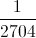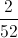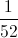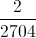Explanation:

Probability of drawing first ace of hearts: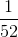Probability of drawing second ace of hearts:Multiply both probabilities by each other: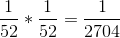### Example Question #1 : How To Identify Independent Variables

A fair coin is flipped three times and comes up heads each time. What is the probability that the fourth toss will also come up heads?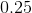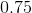Explanation:

Remember, no matter what the previous trials' results, the probability of a head (or tail) does not change frombecause each trial is independent of the others.

### Example Question #1 : How To Identify Independent Variables

What is the probability that a person will flip a coin and land on heads eight times in a row? Assume that the coin is fair and can either land on heads or tails.

1 out of 512

1 out of 256

It is not possible to flip a coin and land on heads ten times in a row.

1 out of 128

1 out of 64

1 out of 256

Explanation:

In this problem, it is important to identify that flipping a coin is an independent event. One coin flip does not affect the next coin flip. In each flip, there is a 50% chance of landing on heads and a 50% chance of landing on tails. By multiplying the probabilities with each other eight times, we get the following: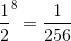### Example Question #1 : How To Identify Independent Variables

A dice is rolled four times in a row. What is the probability that every one of the four rolls will result in a result greater than 2? Assume the dice is fair and has six sides.

4 out of 6

25 out of 30

16 out of 81

2 out of 3

75 out of 80

16 out of 81

Explanation:

In this problem, each roll of the dice is independent from the previous roll. The probability of obtaining a result of greater than 2 on a single roll is 4/6 or 2/3 (since 3, 4, 5, and 6 are all greater than 2). In order to find the probability that this occurs four times in a row, we must multiply the following: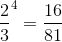### Example Question #1 : How To Identify Independent Variables

Four people are playing a card game and each of them has their own 52-card deck that has been randomly shuffled.

What is the probability that all four of the individuals will draw a face card at random?

12 out of 52

1 out of 52

6000 out of 100000

81 out of 28561

9 out of 52

81 out of 28561

Explanation:

Since each deck of cards is separate and only one card is being drawn from each deck, the events are independent of each other. The probability of drawing a single face card (Jacks, Queens, or Kings) from a deck of cards is 12/52. We can multiply the probabilities as follows: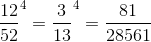### Example Question #1 : Independent Random Variable Combination

A dice is rolled five times in a row. Each time, a 6 is rolled. What is the probability that a 6 will be rolled on the sixth roll? Assume that the dice is fair and has six sides.The answer cannot be determined with the information given.Explanation:

Regardless of the previous results of the dice rolls, the probability of each individual dice roll remains the same. Each time the dice is rolled, there is a 1/6 chance that it will land on a specific number. Therefore, there is a 1/6 chance that the dice will roll a 6 on the sixth roll.

### Example Question #121 : Statistical Patterns And Random Phenomena

A coin is tossed 100 times and each time, the coin lands on tails. What is the expected probability of the coin landing on tails if it is rolled an infinite number of times? Assume that the coin is fair and has two sides - heads and tails.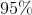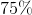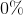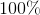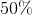Explanation:

According to the Law of Large Numbers, the probability of the coin landing on tails after being rolled an infinite number of times is equal to the probability of the coin landing on tails in an individual roll: 50%.

### Example Question #1 : How To Identify Independent Variables

A student is conducting different experiments that include the rain fall totals in his town, the change in temperature, the tidal levels on the beach, and the humidity.  These will all be measured over the next couple of months to figure out the current trends.  What would the independent event be in these experiments?

Rain fall in inches

Temperature in degrees

Humudiity percentage

Time in hours

Tidal levels in feet

Time in hours

Explanation:

Time is the independent variable as time is not affected by the other variables but the other variables all change as time lapses.

### Example Question #1 : Dependence And Independence

Which of the following represents a continuous variable?

Two of these.

The number of fish in aquariums.

None of these.

The concentration of DNA in a liquid sample.

The weight of footballs.

Two of these.

Explanation:

A continuous variable may assume an infinite number of different values between two given points while a discrete variable may only assume a specific number of specific values between two given points. Given this information, it becomes apparent that the concentration of DNA in a liquid sample and the weight of footballs are both continuous variables.

### Example Question #1 : How To Identify Dependent Variables

A hair care company is testing a new shampoo formula for its ability to keep hair clean for longer thanks to a new chemical compound XYZ that should limit oil production.

To test the appropriate strength of the shampoo, they are testing several concentrations of chemical XYZ to and measuring the amount of oil on the participant's scalp after 24 hours. The color of the shampoo will be randomly changed to keep the participants from knowing what shampoo is which strength.

Which of the following is the Dependant variable?

Amount of Oil on Participant's Scalp

Gender

Length of Participant Hair

Shampoo Color

Concentration of Chemical XYZ in shampoo

Amount of Oil on Participant's Scalp

Explanation:

The Independent variable is what the experimenters are directly manipulating-- here, that is the strength of the Chemical XYZ.

The Dependent variable is the variable that changes based on the Independent variable-- in this case, it is the amount of oil on participants' scalps.

← Previous 1

### All AP Statistics Resources